﻿ 配电网对分布式光伏的接纳能力分析 Analysis of the Acceptance Ability of Distribution Network to Distributed PV

Advances in Energy and Power Engineering
Vol.05 No.06(2017), Article ID:23105,10 pages
10.12677/AEPE.2017.56022

Analysis of the Acceptance Ability of Distribution Network to Distributed PV

Xiaoguang Qi1, Peng Xi1, Hong Liu2, Zhengyang Xu2

1Economic Research Institute, State Grid Hebei Electric Power Company, Shijiazhuang Hebei

2Key Laboratory of Smart Grid of Ministry of Education (Tianjin University), Tianjin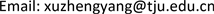Received: Nov. 28th, 2017; accepted: Dec. 14th, 2017; published: Dec. 21st, 2017ABSTRACT

In this paper, the impact of distributed photovoltaic (PV) access on the power quality and fault current of distribution network is studied. First of all, the constant power output of photovoltaic power model is adopted and the influence of the access of distributed photovoltaic on distribution network voltage deviation and voltage harmonic are analyzed; Secondly, the change of fault current is analyzed considering fault at the upstream, downstream and adjacent feeder of the distributed PV access location, respectively; Finally, practical example is calculated based on above theoretical analysis, and the maximum penetration of PV is calculated in comprehensive consideration of power quality and existing protection setting value.

Keywords:Distribution Network, Distributed PV, Impact Analysis, Maximum Penetration

1国网河北省电力公司经济技术研究院，河北 石家庄

2智能电网教育部重点实验室(天津大学)，天津1. 引言

2. 分布式光伏接入对配电网电能质量的影响

2.1. 分布式光伏接入后对配电网的电压偏差的影响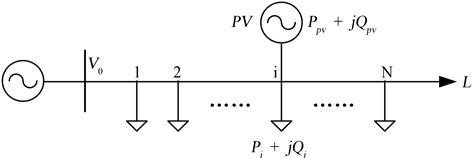Figure 1. Multi node constant power distribution network

$\begin{array}{c}\Delta {{U}^{\prime }}_{i}=\frac{{V}_{i}-{V}_{N}}{{V}_{N}}=\frac{{V}_{0}-\Delta {V}_{0,i}-{V}_{N}}{{V}_{N}}\\ =\frac{{V}_{0}}{{V}_{N}}-\frac{i\left(2N-i+1\right)}{2}×\frac{{P}_{l}×R+{Q}_{l}×X}{{V}_{N}^{2}}-1\end{array}$ (1)

$\Delta {V}_{pvj}=\left\{\begin{array}{l}-\frac{j\left(R{P}_{pv}+X{Q}_{pv}\right)}{{V}_{N}},j\in \left[1,i\right]\\ -\frac{i\left(R{P}_{pv}+X{Q}_{pv}\right)}{{V}_{N}},j\in \left[i+1,N\right]\end{array}$ (2)

$\Delta {V}_{j}=\left\{\begin{array}{l}\frac{j\left(2N-j+1\right)}{2}×\frac{R{P}_{l}+X{Q}_{l}}{{V}_{N}}-\frac{j\left(R{P}_{pv}+X{Q}_{pv}\right)}{{V}_{N}},j\in \left[1,i\right]\\ \frac{j\left(2N-j+1\right)}{2}×\frac{R{P}_{l}+X{Q}_{l}}{{V}_{N}}-\frac{i\left(R{P}_{pv}+X{Q}_{pv}\right)}{{V}_{N}},j\in \left[i+1,N\right]\end{array}$ (3)

${V}_{j}=\left\{\begin{array}{l}{V}_{0}-\frac{j\left(2N-j+1\right)}{2}×\frac{R{P}_{l}+X{Q}_{l}}{{V}_{N}}+\frac{j\left(R{P}_{pv}+X{Q}_{pv}\right)}{{V}_{N}},j\in \left[1,i\right]\\ {V}_{0}-\frac{j\left(2N-j+1\right)}{2}×\frac{R{P}_{l}+X{Q}_{l}}{{V}_{N}}+\frac{i\left(R{P}_{pv}+X{Q}_{pv}\right)}{{V}_{N}},j\in \left[i+1,N\right]\end{array}$ (4)

$\Delta {U}_{j}=\left\{\begin{array}{l}\frac{{V}_{0}}{{V}_{N}}-\frac{j\left(2N-j+1\right)}{2}×\frac{R{P}_{l}+X{Q}_{l}}{{V}_{N}^{2}}+\frac{j\left(R{P}_{pv}+X{Q}_{pv}\right)}{{V}_{N}^{2}}-1,j\in \left[1,i\right]\\ \frac{{V}_{0}}{{V}_{N}}-\frac{j\left(2N-j+1\right)}{2}×\frac{R{P}_{l}+X{Q}_{l}}{{V}_{N}^{2}}+\frac{i\left(R{P}_{pv}+X{Q}_{pv}\right)}{{V}_{N}^{2}}-1,j\in \left[i+1,N\right]\end{array}$ (5)

2.2. 新能源系统接入对谐波电压畸变量的影响

2.2.1. 分布式光伏系统接入容量对谐波电压畸变量的影响

$|{I}_{pv}|=|\frac{{P}_{pv}}{{V}_{pv}\ast dp{f}_{pv}}|$ (6)

$\begin{array}{c}\Delta {V}_{h}=|{V}_{h,2}|-|{V}_{h,1}|=\sqrt{{r}^{2}+{h}^{2}{x}^{2}}\ast \left(|{I}_{pvh}|G+|{I}_{lh}|L\right)-L\ast \sqrt{{r}^{2}+{h}^{2}{x}^{2}}\ast |{I}_{lh}|\\ =\sqrt{{r}^{2}+{h}^{2}{x}^{2}}*|{I}_{pvh}|G>0\end{array}$ (7)

2.2.2. 分布式光伏系统接入位置对谐波电压畸变量的影响

1) 分布式光伏系统接入线路 ${G}_{1}$ 之前 $Z$ ( $Z<{G}_{1}$ )位置的谐波电压幅值可表示为： $|{V}_{h}|=|\left(r+jhx\right)\ast Z|\ast |{I}_{pvh}+{I}_{lh}|=Z\sqrt{{r}^{2}+{h}^{2}{x}^{2}}\ast \left(|{I}_{pvh}|+|{I}_{lh}|\right)$ 。由于分布式光伏系统采用电压控制的运行模式，则 ${V}_{pv}$ 基本保持不变， ${P}_{pv}$ 不变时，由方程式(2)可知，在分布式光伏系统的接入节点分别为 ${G}_{1}$${G}_{2}$ 时， $|{I}_{pvh}|$ 几乎相同。而 $G$ 越大，线路末端节点电压 $V$ 就越高，在分布式光伏系统的接入节点为 ${G}_{2}$ 时的 $|{I}_{lh}|$ 比接入节点为 ${G}_{1}$ 时的 $|{I}_{lh}|$ 小。因此，随着接入位置 $G$ 的增大，分布式光伏系统接入点 ${G}_{1}$ 之前 $Z$ ( $Z<{G}_{1}$ )位置的谐波电压幅值 $|{V}_{h}|$ 减小，谐波电压畸变水平降低。

2) 分布式光伏系统接入线路 ${G}_{2}$ 之后 $Z$ ( $Z>{G}_{2}$ )位置的谐波电压幅值可表示为：当分布式光伏系统接入位置为 ${G}_{1}$ 时， $|{V}_{h\left({G}_{1}\right)}|=\sqrt{{r}^{2}+{h}^{2}{x}^{2}}\ast \left[{G}_{1}\left(|{I}_{pvh}|+|{I}_{lh}|\right)+\left(Z-{G}_{1}\right)|{I}_{lh}|\right]$ ；当分布式光伏系统接入位置为 ${G}_{2}$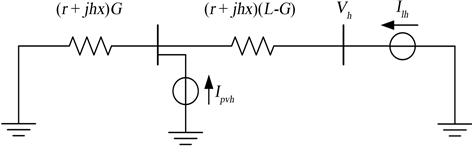Figure 2. Distribution network for harmonic analysis

3. 分布式光伏接入对配电网电流保护的影响

${I}_{k,R1}=\frac{{E}_{s}}{a{Z}_{1-2}}$ (8)

$\left(\frac{1}{{Z}_{L}}+\frac{1}{\left(1-a\right){Z}_{1-2}+{Z}_{2-3}}\right)\stackrel{˙}{U}=\left(\frac{S}{\stackrel{˙}{U}}\right)$ (9)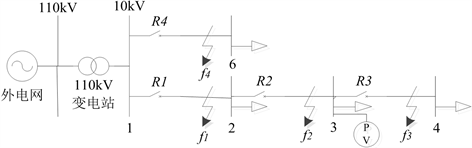Figure 3. Distribution network for relaying protection analysis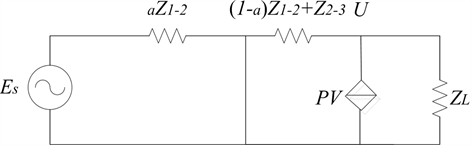Figure 4. Equivalent circuit diagram for short circuit at f1

$\left(1+\frac{|{Z}_{L}|}{\left(1-a\right)|{Z}_{1-2}|+|{Z}_{2-3}|}\right){U}^{2}=P|{Z}_{L}|\mathrm{cos}\beta$ (10)

$U=\sqrt{\frac{P{R}_{L}\left(\left(1-a\right)|{Z}_{1-2}|+|{Z}_{2-3}|\right)}{\left(1-a\right)|{Z}_{1-2}|+|{Z}_{2-3}|+|{Z}_{L}|}}$ (11)

${I}_{k,R2}=\frac{U}{\left(1-a\right)|{Z}_{1-2}|+|{Z}_{2-3}|}=\sqrt{\frac{P{R}_{L}}{{\left(\left(1-a\right)|{Z}_{1-2}|+|{Z}_{2-3}|\right)}^{2}+|{Z}_{L}|\left(\left(1-a\right)|{Z}_{1-2}|+|{Z}_{2-3}|\right)}}$ (12)

4. 算例分析

4.1. 考虑电能质量的分布式光伏准入容量

4.1.1. 电压偏差

4.1.2. 电压谐波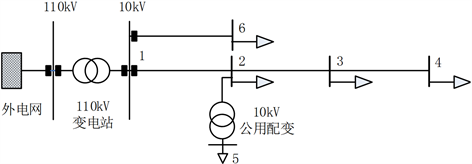Figure 5. A typical distribution networkTable 1. Node voltage deviation when the PV capacity changesTable 2. Node voltage deviation when the PV location changesTable 3. Node voltage deviation when the PV capacity changes

4.2. 考虑电流保护的分布式光伏准入容量Table 4. Node voltage deviation when the PV location changesTable 5. Feeder current protection setting value without considering distributed PVTable 6. Short circuit current effective value of each protection when the PV capacity changesTable 7. Short circuit current effective value of each protection when the PV location changes

4.3. 综合考虑电能质量与电流保护的分布式光伏准入容量

5. 结论

Analysis of the Acceptance Ability of Distribution Network to Distributed PV[J]. 电力与能源进展, 2017, 05(06): 143-152. http://dx.doi.org/10.12677/AEPE.2017.56022

1. 1. 黄炜, 刘健. 分布式光伏电源极端可接入容量极限研究[J]. 电力系统保护与控制, 2015, 43(2): 22-28.

2. 2. 夏成军, 崔弘. 考虑静态安全约束的分布式电源准入容量计算[J]. 电网技术, 2009, 33(16): 96-100..

3. 3. 刘健, 同向前. 考虑过电压因素时分布式光伏电源的准入容量[J]. 电力系统保护与控制, 2014, 42(6): 45-51..

4. 4. 陈祥鹏. 光伏发电并网对配电网电压分布影响的研究[D]: [硕士学位论文]. 淄博: 山东理工大学, 2015.

5. 5. 苏小玲, 韩民晓. 配电网中分布式电源最大准入容量分析[J]. 电网技术, 2012, 36(10): 87-92.

6. 6. 陈玉玺. 分布式电源谐波对配电网的影响研究[D]: [硕士学位论文]. 北京: 北京交通大学, 2016.

7. 7. 管晟超, 任明珠, 谭佳, 孙诗航, 程浩忠. 基于谐波约束的配电网光伏最大准入容量计算[J]. 电网与清洁能源, 2016, 32(2): 89-95.

8. 8. 黄巍, 吴俊勇, 鲁思棋, 郝亮亮. 电压偏差和谐波约束下配网光伏最大渗透率评估[J]. 电力系统保护与控制, 2016, 44(6): 49-55.

9. 9. 李斌, 袁越. 光伏并网发电对保护及重合闸的影响与对策[J]. 电力自动化设备, 2013, 33(4): 12-17.